# Why going for Hamilton Monte Carlo

• Since decades, probability scientists are continuously improving algorithms to draw random samples from pdf known up to a normalizing constant: $\pi(\theta) = \frac{f(\theta)}{\int_\theta f(\theta)d\theta}$ ( $$f(\theta)$$ being a positive measure.)

Especially convenient for Bayesians $\pi(\theta) \propto \mathrm{prior}(\theta) \times \mathrm{Likelihood}(\theta)$ but also useful for frequentists working on latent class models with SEM based algorithms.

• Unleashed Bayesian modelers tend to develop adhoc complex models but at the same time wish to rely effortlessly on available inference toolboxes.

• Common softwares’ (BUGS, JAGS etc.) capabilities become rapidly saturated and the MCMC burn-in and cruising phases may take an unacceptably long while (because standard MCMC provide strongly correlated visits of the target distribution) : need for efficient algorithms (with less correlated trajectories such as the ones obtained from Piecewise Deterministic Markov Processes) as well as quick inference (recourse to C++).

• A new class of gradient-based MC algorithms is becoming incredibly fashionable in many domains of Applied Stats.

• Stan is a well documented statistical language, supported by many big names of the statistical community .

A word of warning:

He who loves practice without theory is like the sailor who boards ship without a rudder and compass and never knows where he may be cast, L. Da Vinci

…, although most friends of mine (with the exception of some Britons, maybe) would go and sail, blindly relying on modern GPS.

# Trying to see farther by standing on giants’shoulders:

Much of the following material has been stolen from:

# Hamiltonian Agenda

• Part 1 : a bit of theory

1. At the beginning was Physics: Hamiltonian dynamics

2. From Physics to Maths: Hamiltonian properties

3. From Maths to Stats: Hamiltonian MCMC

• Part 2 : How to launch and run Stan (on a cherry tree)

• Part 3 : Going further with Stan

# Physics

## Gravity : Newton ’s falling appleWith the dot notation for derivatives:

\begin{align} \vec{f} & =m\vec{\gamma}\\ mg & =m\ddot{x}\\ mg\dot{x} & =m\ddot{x}\dot{x}\\ 0 & =\frac{d}{dt}(-mgx+\frac{1}{2}mv^{2}) \end{align}

$\underset{\text{Energy}}{\underbrace{H(v,x)}}=\underset{\text{Potential}% }{\underbrace{U(x)}}+\underset{\text{Cinetic}}{\underbrace{K(v)}}$

## Coiled spring : oscillations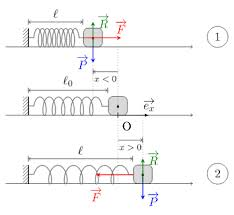\begin{align*} f & =m\gamma\\ -kx & =m\ddot{x}\\ -kx\dot{x} & =m\ddot{x}\dot{x}\\ 0 & =\frac{d}{dt}(\frac{1}{2}mx^{2}+\frac{1}{2}mv^{2}) \end{align*}

$\underset{\text{Energy}}{\underbrace{H(v,x)}}=\underset{\text{Potential}% }{\underbrace{U(x)}}+\underset{\text{Cinetic}}{\underbrace{K(v)}}$

## More complex systems in physics obbey Hamilton equations

Usually, the total energy is written as:

$\underset{\text{Energy}}{\underbrace{H(q,p)}}=\underset{\text{Potential}% }{\underbrace{U(q)}}+\underset{\text{Cinetic}}{\underbrace{K(p)}}$

$$p$$ : generalized momentum vector $$\in\mathbb{R}^{d}$$

$$q$$ : generalized position vector $$\in\mathbb{R}^{d}$$

\begin{align} \frac{dq}{dt} & =\frac{\partial H(q,p)}{\partial p}\\ \frac{dp}{dt} & =-\frac{\partial H(q,p)}{\partial q} \end{align}

# From Physics to Maths: Hamiltonian properties

What are the properties of a function $$H(p,q)$$… such that:

\begin{align*} \frac{dq}{dt} & =\frac{\partial H(q,p)}{\partial p}\\ \frac{dp}{dt} & =-\frac{\partial H(q,p)}{\partial q}% \end{align*}

More globally, in just a single equation:

$\frac{dz}{dt}=J\times\nabla H$

with $$z=\left(\begin{array}[c]{c} q\\ p \end{array} \right)$$, $$J=\left(\begin{array}[c]{cc} 0_{d\times d} & 1_{d\times d}\\ -1_{d\times d} & 0_{d\times d} \end{array} \right)$$

## Hamiltonian property 1 : Reversibility

The mapping $$\mathcal{T}_{s}$$ : $$\left( t,\left( \begin{array}[c]{c} q(t)\\ p(t) \end{array} \right) \right) \longmapsto\left( t+s,\left( \begin{array}[c]{c} q(t+s)\\ p(t+s) \end{array}\right)\right)$$ is one-to-one, thus reversible.

If $$H(q,p)=U(q)+K(p)$$ with $$K(p)=K(-p)$$, $$\mathcal{T}_{-s}$$ is obtained by the sequence of operations $$(p\rightarrow-p),\mathcal{T}_{s},(p\rightarrow-p)$$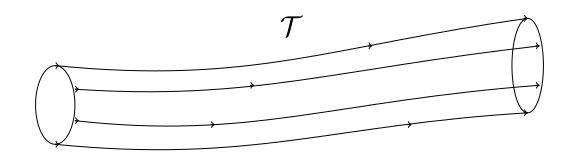## Hamiltonian property 2 : Conservation of $$H$$ along the trajectories of $$\mathcal{T}$$

\begin{align*} \frac{dH}{dt} & =\left( \frac{\partial H(q,p)}{\partial p}\right) \frac {dp}{dt}+\left( \frac{\partial H(q,p)}{\partial q}\right) \frac{dq}{dt}\\ & =\frac{\partial H(q,p)}{\partial p}\left( -\frac{\partial H(q,p)}{\partial q}\right) +\frac{\partial H(q,p)}{\partial q}\left( \frac{\partial H(q,p)}{\partial p}\right) \\ & =0 \end{align*}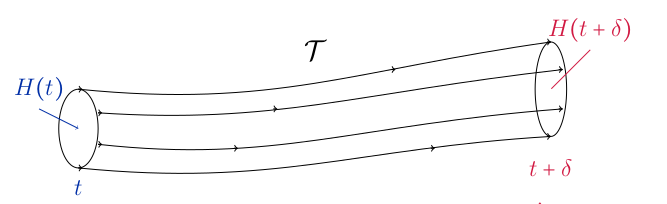## Hamiltonian property 3 : Volume preservation along the trajectories of $$\mathcal{T}$$

A vector field $$\mathcal{T}$$ with $$0$$ divergence preserves volume:

\begin{align*} \Delta\mathcal{T} & =\left( \frac{\partial}{\partial p}\right) \frac {dp}{dt}+\left( \frac{\partial}{\partial q}\right) \frac{dq}{dt}\\ & =\frac{\partial}{\partial p}\left( -\frac{\partial H(q,p))}{\partial q}\right) +\frac{\partial}{\partial q}\left( \frac{\partial H(q,p)}{\partial p}\right) \\ & =-\left( \frac{{\partial^{2}}H(q,p)}{{\partial p}{\partial q}}\right) +\left( \frac{{\partial^{2}}H(q,p)}{{\partial p}{\partial q}}\right) =0 \end{align*}

i.e. preservation of pdf by a change of variables since the determinant of the Jacobian matrix $$J_{\delta}$$ for a change of coordinates $$\mathcal{T}_{\delta}$$ from $$s$$ to $$s+\delta$$ will be $$1$$!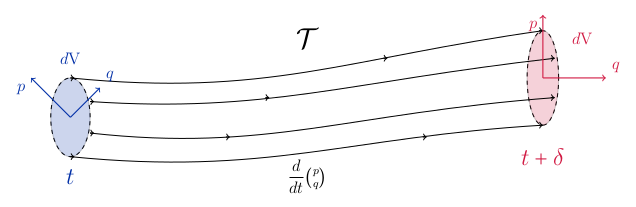# Numerical integration along the trajectories of $$\mathcal{T}$$

How to practically evaluate $$\mathcal{T}_{\delta}\left(\begin{array}[c]{c} q(t)\\ p(t) \end{array}\right)$$ for a small $$\delta$$?

## Euler’s discretisation

\begin{align*} \left(\begin{array}[c]{c} q(t+\delta)\\ p(t+\delta) \end{array}\right) & \approx\left(\begin{array}[c]{c} q(t)\\ p(t) \end{array}\right) +\delta\left(\begin{array}[c]{c} \frac{dq(t)}{dt}\\ \frac{dp(t)}{dt} \end{array}\right) \\ & =\left(\begin{array}[c]{c} q(t)+\delta\frac{\partial H(q,p)}{\partial p}\\ p(t)-\delta\frac{\partial H(q,p)}{\partial q} \end{array}\right) \end{align*}

if $$H(q,p)=U(q)+K(p)$$ and $$K(p)=\frac{1}{2}p^{\prime}M^{-1}p$$

\begin{align*} \left(\begin{array}[c]{c} q(t+\delta)\\ p(t+\delta) \end{array} \right) & \approx\left( \begin{array}[c]{c} q(t)+\delta\frac{\nabla K(p)}{\partial p}\\ p(t)-\delta\frac{\nabla U(q)}{\partial q} \end{array} \right) \\ & \approx\left( \begin{array}[c]{c} q(t)+\delta\times M^{-1}p(t)\\ p(t)-\delta\times\frac{\partial U}{\partial q}(q(t)) \end{array} \right) \end{align*}

Euler’s scheme may encounter problems…Let’s examine the example reproduced from FIG 5.1 of Neal with $$H(q,p)=q^{2}/2+p^{2}/2$$ whose trajectories are circles. (The initial state is $$q=0,p=1$$. $$L=20$$ steps)

Eulerstep = function(epsilon, L, current_q, current_p) {
Q <- current_q
P <- current_p
for (i in 1:L) {
q = current_q + 1 * current_p * epsilon
p = current_p - 1 * epsilon * current_q
current_p = p
current_q = q
Q = c(Q, q)
P = c(P, p)
}
return(list(P = P, Q = Q))
}
angle = seq(0, 2 * pi, length.out = 1000)
plot(cos(angle), sin(angle), lwd = 4, type = 'l', lty = 3, las = 1,
xlab = "position q",ylab = "position q", xlim = c(-2, 2), ylim = c(-2, 2))
xy = Eulerstep(epsilon = 0.3, L = 20, current_q = 0, current_p = 1)
lines(xy$Q, xy$P, type = "b", pch = 19, col = 'red')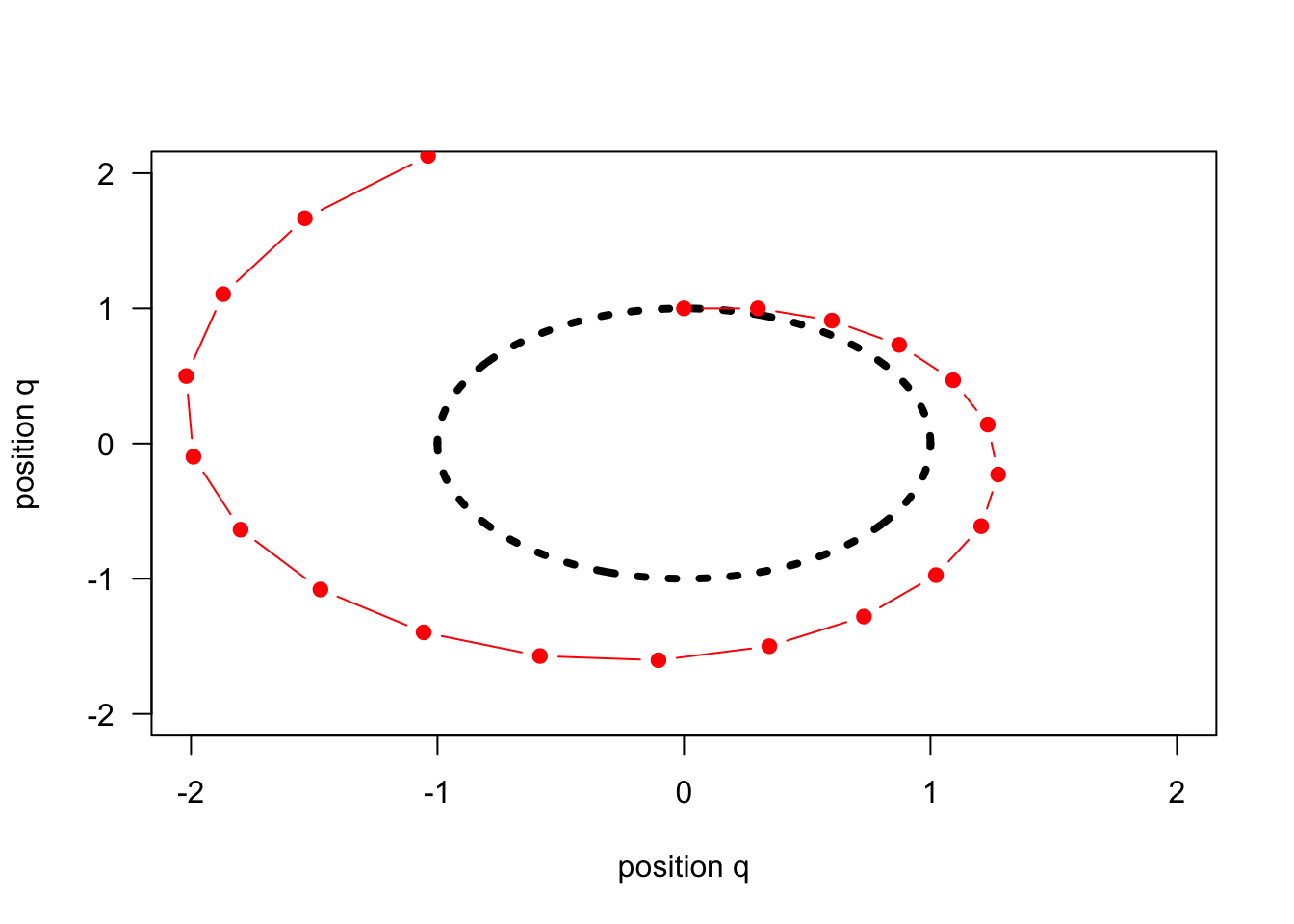## Euler’s modified discretisation

There are ways to improve the discretisation, for instance Euler’s shear transformation works as follows:

\begin{align*} q(t+\delta) & \approx q(t)+\delta\times M^{-1}p(\mathbf{t})\\ p(t+\delta) & \approx p(t)-\delta\times\frac{\partial U}{\partial q}(q(\mathbf{t+\delta})) \end{align*}
EulerModifstep = function(epsilon, L, current_q, current_p){
Q = current_q
P = current_p
for (i in 1:L) {
current_q = current_q + 1 * current_p * epsilon
current_p = current_p - 1 * epsilon * current_q
Q = c(Q, current_q)
P = c(P, current_p)
}
return(list(P = P, Q = Q))
}
plot(cos(angle), sin(angle), lwd = 4, type = 'l', lty = 3, las = 1,
xlab = "position q", ylab = "position q", xlim = c(-2, 2), ylim = c(-2, 2))
xymod = EulerModifstep(epsilon = 0.3, L = 20, current_q = 0, current_p = 1)
lines(xymod$Q, xymod$P, col = 'blue', type = "b", pch = 19)